Monday, March 30, 2020
Home > CBSE Class 12 > NCERT Solutions for Class 12 Maths Integration Exercise 7.3

# NCERT Solutions for Class 12 Maths Integrations

Hi Students, Welcome to Amans Maths Blogs (AMB). In this post, you will get the NCERT Solutions for Class 12 Maths Integration Exercise 7.3.

NCERT Solutions for class 12 is highly recommended by the experienced teacher for students who are going to appear in CBSE Class 12 and JEE Mains and Advanced and NEET level exams. Here You will get NCERT Solutions for Class 12 Maths Integration Exercise 7.3 of all questions given in NCERT textbooks of class 12 in details with step by step process.

CBSE Class 12th is an important school class in your life as you take some serious decision about your career. And out of all subjects, Maths is an important and core subjects. So CBSE NCERT Solutions for Class 12th Maths is major role in your exam preparation as it has detailed chapter wise solutions for all exercise. This NCERT Solutions can be downloaded in PDF file. The downloading link is given at last.

The PDF books of NCERT Solutions for Class 12 are the first step towards the learning and understanding the each sections of Maths, Physics, Chemistry, Biology as it all help in engineering medical entrance exams. To solve it, you just need to click on download links of NCERT solutions for class 12.

NCERT Solutions for Class 12 Maths are not only the solutions of Maths exercise but it builds your foundation of other important subjects. Getting knowledge of depth concept of CBSE Class 12th Maths like Algebra, Calculus, Trigonometry, Coordinate Geometry help you to understand the concept of Physics and Physical Chemistry.

As we know that all the schools affiliated from CBSE follow the NCERT books for all subjects. You can check the CBSE NCERT Syllabus. Thus, NCERT Solutions helps the students to solve the exercise questions as given in NCERT Books

## NCERT Solutions for Class 12 Maths Integration Exercise 7.3

Find the integrals of the functions in Exercises 1 to 22:

NCERT Solutions for Class 12 Maths Integration Exercise 7.3: Ques No 1.

sin2 (2x + 5)

[Integrate sin2 (2x + 5)]

NCERT Solutions:NCERT Solutions for Class 12 Maths Integration Exercise 7.3: Ques No 2.

sin 3x cos 4x

[Integrate sin 3x cos 4x]

NCERT Solutions: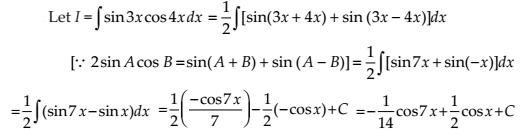NCERT Solutions for Class 12 Maths Integration Exercise 7.3: Ques No 3.

cos 2x cos 4x cos 6x

[Integrate cos 2x cos 4x cos 6x]

NCERT Solutions: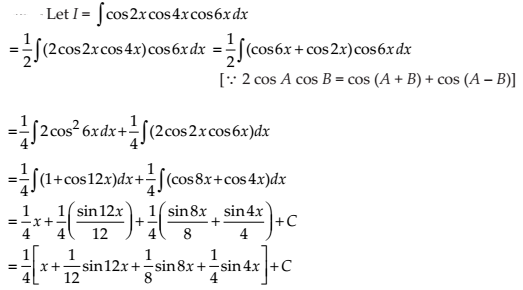NCERT Solutions for Class 12 Maths Integration Exercise 7.3: Ques No 4.

sin3 (2x + 1)

[Integrate sin3 (2x + 1)]

NCERT Solutions:NCERT Solutions for Class 12 Maths Integration Exercise 7.3: Ques No 5.

sin3 x cos3 x

[Integrate sin3 x cos3 x]

NCERT Solutions: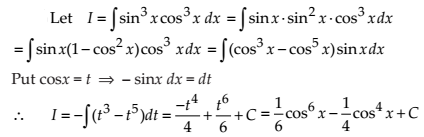NCERT Solutions for Class 12 Maths Integration Exercise 7.3: Ques No 6.

sin x sin 2x sin 3x

[Integrate sin x sin 2x sin 3x]

NCERT Solutions:NCERT Solutions for Class 12 Maths Integration Exercise 7.3: Ques No 7.

sin 4x sin 8x

[Integrate sin 4x sin 8x]

NCERT Solutions: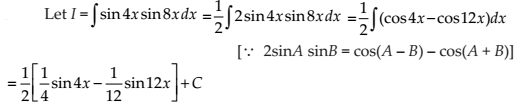NCERT Solutions for Class 12 Maths Integration Exercise 7.3: Ques No 8.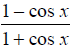[Integrate (1 – cos x)/(1 + cos x)]

NCERT Solutions:NCERT Solutions for Class 12 Maths Integration Exercise 7.3: Ques No 9.[Integrate cos x/(1 + cos x)]

NCERT Solutions: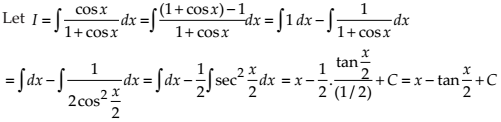NCERT Solutions for Class 12 Maths Integration Exercise 7.3: Ques No 10.

sin4 x

[Integrate sin4 x]

NCERT Solutions:NCERT Solutions for Class 12 Maths Integration Exercise 7.3: Ques No 11.

cos4 2x

[Integrate cos4 2x]

NCERT Solutions: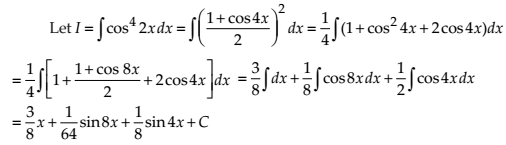NCERT Solutions for Class 12 Maths Integration Exercise 7.3: Ques No 12.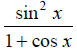[Integrate sin2 x / (1 + cos x)]

NCERT Solutions:NCERT Solutions for Class 12 Maths Integration Exercise 7.3: Ques No 13.[Integrate (cos 2x – cos 2 alpha) / (cos x – cos alpha)]

NCERT Solutions: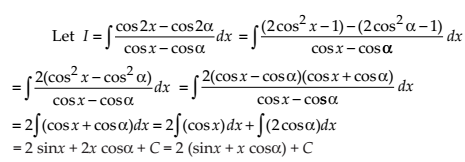NCERT Solutions for Class 12 Maths Integration Exercise 7.3: Ques No 14.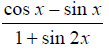[Integrate (cos x – sin x) / (1 + sin 2x)]

NCERT Solutions:NCERT Solutions for Class 12 Maths Integration Exercise 7.3: Ques No 15.

tan3 2x sec 2x

[Integrate tan3 2x sec 2x]

NCERT Solutions:NCERT Solutions for Class 12 Maths Integration Exercise 7.3: Ques No 16.

tan4 x

[Integrate tan4 x]

NCERT Solutions:NCERT Solutions for Class 12 Maths Integration Exercise 7.3: Ques No 17.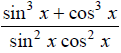[Integrate (sin3 x + cos3 x)/sin2 x cos2 x]

NCERT Solutions: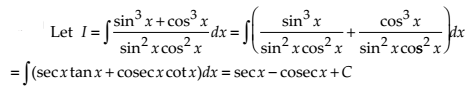NCERT Solutions for Class 12 Maths Integration Exercise 7.3: Ques No 18.[Integrate (cos 2x + 2sin2 x)/cos2 x]

NCERT Solutions:NCERT Solutions for Class 12 Maths Integration Exercise 7.3: Ques No 19.[Integrate 1/(sin x cos3 x)]

NCERT Solutions:NCERT Solutions for Class 12 Maths Integration Exercise 7.3: Ques No 20.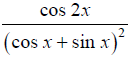[Integrate cos 2x/(cos x + sin x)2]

NCERT Solutions:NCERT Solutions for Class 12 Maths Integration Exercise 7.3: Ques No 21.

sin–1 (cos x)

[Integrate sin inverse  (cos x)]

NCERT Solutions:NCERT Solutions for Class 12 Maths Integration Exercise 7.3: Ques No 22.[Integrate 1 / (cos (x – a)cos (x – b))]

NCERT Solutions: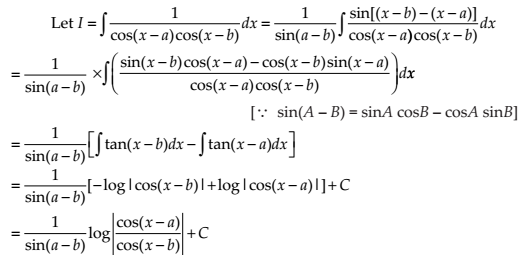Choose the correct answer in Exercises 23 and 24.

NCERT Solutions for Class 12 Maths Integration Exercise 7.3: Ques No 23.equals to

[Integrate (sin2 x – cos2 x) / (sin2 x cos2 x)]

(A) tan x + cot x + C

(B) tan x + cosec x + C

(C) – tan x + cot x + C

(D) tan x + sec x + C

NCERT Solutions:NCERT Solutions for Class 12 Maths Integration Exercise 7.3: Ques No 23.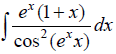equals to

[Integrate (ex)(1 + x) / (cos2 (ex))]

(A) – cot (exx) + C

(B) tan (xex) + C

(C) tan (ex) + C

(D) cot (ex) + C

NCERT Solutions: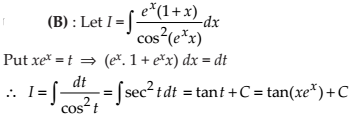error: Content is protected !!